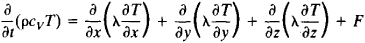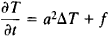# Heat Equation

(redirected from Particle diffusion)

## heat equation

[′hēt i‚kwā·zhən]
(thermodynamics)
A parabolic second-order differential equation for the temperature of a substance in a region where no heat source exists: ∂ t /∂τ = (kc)(∂2 t /∂ x 2+ ∂2 t /∂ y 2+ ∂ t 2/∂ z 2), where x, y, and z are space coordinates, τ is the time, t (x,y,z, τ) is the temperature, k is the thermal conductivity of the body, ρ is its density, and c is its specific heat; this equation is fundamental to the study of heat flow in bodies. Also known as Fourier heat equation; heat flow equation.

## Heat Equation

a parabolic partial differential equation that describes the process of propagation of heat in a continuous medium, such as a gas, liquid, or solid. It is the basic equation in the mathematical theory of thermal conductivity.

The heat equation expresses the heat balance for a small element of volume of the medium; heat gains from sources and heat losses through the surface of the element are taken into account for heat transport by conduction. The equation has the following form for an isotropic nonhomogeneous medium:Here, ρ is the density of the medium; cv is the specific heat of the medium at constant volume; t is time; x, y, and z are space coordinates; T = T(x, y, z, t) is the temperature, which is calculated by means of the heat equation; λ is the coefficient of thermal conductivity; and F = F(x, y, z, t) is the specified density of the heat sources. The magnitudes of ρ cv, and λ depend on the coordinates and, generally speaking, on the temperature. For an anisotropic medium, the heat equation contains in place of λ the thermal conductivity tensor λik, where i, k = 1,2,3.

In the case of an istropic homogeneous medium, the heat equation assumes the formwhere Δ is the Laplace operator, a2 = λ/(ρcv) is the coefficient of thermal diffusivity, and f = F/(ρcv). In a stationary state, where the temperature does not vary with time, the heat equation becomes the Poisson equation or, when there are no heat sources, Laplace’s equation ΔT = 0. The basic problems for the heat equation are the Cauchy problem and the mixed boundary value problem (seeBOUNDARY VALUE PROBLEMS).

The heat equation was first studied by J. Fourier in 1822 and S. Poisson in 1835. Important results in the study of the heat equation were obtained by I. G. Petrovskii, A. N. Tikhonov, and S. L. Sobolev.

### REFERENCES

Carslaw, W. S. Teoriia teploprovodnosti. Moscow-Leningrad, 1947.
Vladimirov, V. S. Uravneniia matematicheskoi fiziki. Moscow, 1967.
Tikhonov, A. N., and A. A. Samarskii. Uravneniia matematicheskoi fiziki, 3rd ed. Moscow, 1966.

D. N. ZUBAREV

References in periodicals archive ?
in which [epsilon] is the particle diffusion coefficient, set as an assumption of the exponential probability density function
If the straight line of the plot of qe against t 1/2 passes through the origin, then intra particle diffusion becomes the controlling step.
The mechanism behind such behaviour of MG dye onto NOAC is analysed by intra particle diffusion model.
AB] and Sh are soot mass concentration, thermophoretic drift velocity, particle diffusion coefficient, and Sherwood number respectively.
So adsorption diffusion models are developed to explain the film diffusion and/or the process of intra particle diffusion.
If the plot of ln(1-[alpha]) versus time is linear, then adsorption is controlled by particle diffusion and the diffusion of the metal ions onto the adsorbent surface is independent of the extent of adsorption while a non-linear plot indicates adsorbent film-diffusion controlled process, i.
1980), it is likely that two particle diffusion mechanisms were involved (Sivasubramaniam and Talibudeen 1972).
To identify whether seagrass mitigates particle diffusion, we calculated rates of fluorescein dye diffusion inside and outside of grass beds by using video recordings of blobs of fluorescein dye in one two-dimensional vertical plane.
5 [micro]m) This result indicates that particle deposition on the top of moving object is influenced by particle diffusion while the fluid flow convection plays less effect on the particle deposition.
Consistent, seamless measurement was further demonstrated in the raw data, in which diffracted light intensity was plotted against the particle diffusion time.
The change of these profiles is obvious, namely, in the case of no collision the axial velocity profile becomes narrower that is related to the reduction of the particle diffusion in the radial direction caused by the interparticle collisions [4, 5].

Site: Follow: Share:
Open / Close